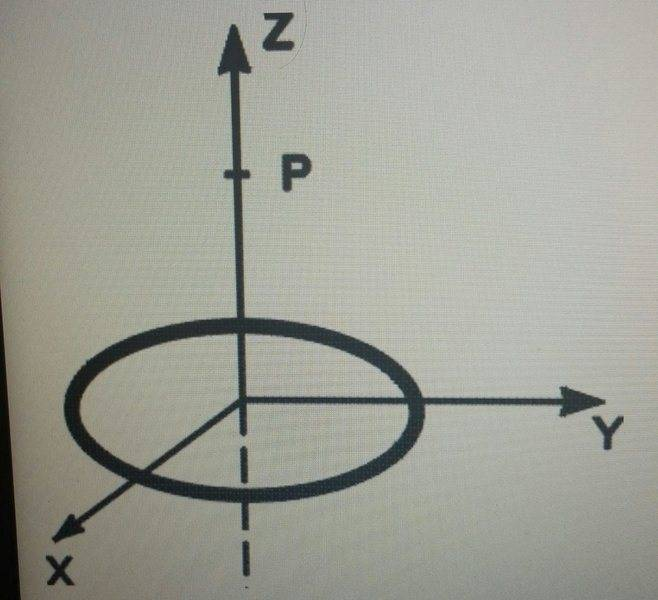# Field due to ring of charge

## Homework Statement

A uniform circular ring of charge Q=4.20 microCoulombs and radius R=1.30 cm is located in the x-y plane, centered on the origin as shown in the figure.

What is the magnitude of the electric field E at point P, located at z=3.70 cm?

I have worked out the problem and got the following formula which I know is correct but when I enter in my numbers it doesn't seem to give me the correct answer, so I'm wondering what I am doing wrong (see below)

E_z = (kQx)/((x^2+R^2)^(3/2))

where k=9x10^9, Q = 4.2*10^-6, x= 0.0130, and R = 0.0370.

## The Attempt at a Solution

I have worked out the problem and got the following formula which I know is correct but when I enter in my numbers it doesn't seem to give me the correct answer, so I'm wondering what I am doing wrong (see below)

E_z = (kQx)/((x^2+R^2)^(3/2))

where k=9x10^9, Q = 4.2*10^-6, x= 0.0130, and z = 0.0370.

So what am I doing wrong? are my numbers above correct?2.316×10^7 N/C

Last edited:

rcgldr
Homework Helper
Looks like you swapped constants. This could be avoided if you restated the equation as

E_z = (kQz)/((z^2+R^2)^(3/2))

R = .0130 m
z = .0370 m
...

Looks like you swapped constants. This could be avoided if you restated the equation as

E_z = (kQz)/((z^2+R^2)^(3/2))

R = .0130 m
z = .0370 m
...

thanks that fixed my problem!

(